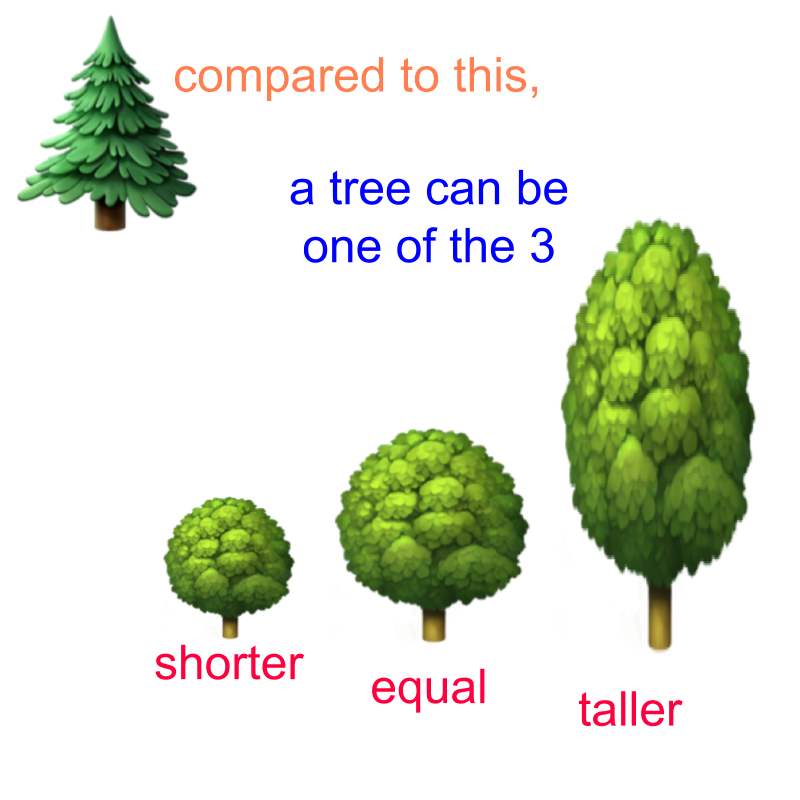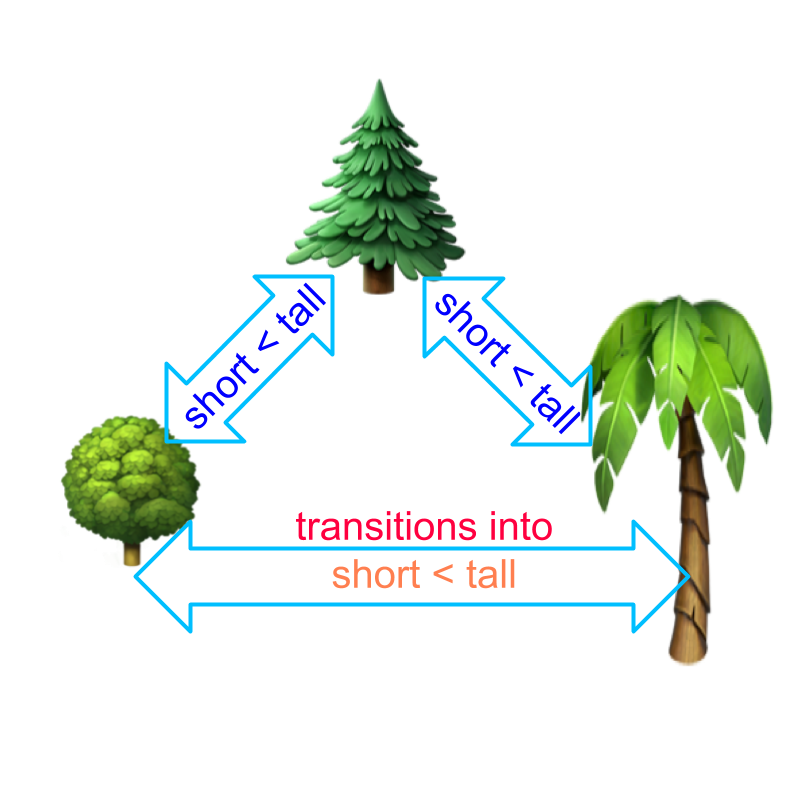maths > algebra

Laws and Properties of Arithmetics : Comparison

what you'll learn...

Overview

Comparison

»  Trichotomy Property
"tri-" means three and "-chotomy" means division-into-parts.
→  Given $a,b\in ℝ$$a , b \in \mathbb{R}$ one and only one of the following is true.
$a$a < b$
$a=b$$a = b$
$a>b$$a > b$

»  Transitivity
"trans-" means across and "-itivity" means to-go or to-pass
→  If $a=b$$a = b$ and $b=c$$b = c$, then it implies $a=c$$a = c$
→  If $a$a < b$ and $b$b < c$, then it implies $a$a < c$
→  If $a>b$$a > b$ and $b>c$$b > c$, then it implies $a>c$$a > c$

three possibilities

Given two real numbers $p$$p$ and $q$$q$, which of the following is correct?Trichotomy Property: For any numbers $p,q\in ℝ$$p , q \in \mathbb{R}$, one and only one of the following is true.
$p$p < q$
$p=q$$p = q$
$p>q$$p > q$

Note: sometimes, $p\le q$$p \le q$ is considered. This specifies, consider pair of numbers $p$$p$ and $q$$q$ such that $p$$p$ is less than or equal to $q$$q$.

It specifies which numbers to choose. That is, if $q$$q$ is 4, then $p$$p$ can be any negative number or $0,1,2,3,4$$0 , 1 , 2 , 3 , 4$. It defines a set of numbers for $p$$p$.

In trichotomy property, for any two numbers (for example, $4$$4$ and $3$$3$), one and only one of the three possibilities is true.

The word "trichotomy" means: Division into three parts.

tri- means three and -chotomy means division-into-parts

pass across

For three real numbers $p,q,r$$p , q , r$, it is given that $p$p < q$ and $q$q < r$, then $p$p < r$Transitivity Property: For three numbers $p,q,r\in ℝ$$p , q , r \in \mathbb{R}$,

•  if $p$p < q$ and $q$q < r$, then $p$p < r$.

•  if $p=q$$p = q$ and $q=r$$q = r$, then $p=r$$p = r$.

•  if $p>q$$p > q$ and $q>r$$q > r$, then $p>r$$p > r$.

Note 1: If $p$p < q$ and $q>r$$q > r$, then the relation between $p$$p$ and $r$$r$ cannot be ascertained.

Note 2: If $p$p < q$ and $q=r$$q = r$, then $q$$q$ can be replaced by $r$$r$ to get $p$p < r$.

The word "transitivity" means: some property passes across from one to another.

trans- means across and -itivity means to-go or to-pass.

summary

LPA - Comparison : Comparison of numbers has Trichotomy and Transitivity properties.

»  Trichotomy Property
"tri-" means three and "-chotomy" means division-into-parts.
→  Given $a,b\in ℝ$$a , b \in \mathbb{R}$ one and only one of the following is true.
$a$a < b$
$a=b$$a = b$
$a>b$$a > b$

»  Transitivity Property
"trans-" means across and "-itivity" means to-go or to-pass
→  If $a=b$$a = b$ and $b=c$$b = c$, then it implies $a=c$$a = c$
→  If $a$a < b$ and $b$b < c$, then it implies $a$a < c$
→  If $a>b$$a > b$ and $b>c$$b > c$, then it implies $a>c$$a > c$

Outline

The outline of material to learn "Algebra Foundation" is as follows.

→   Numerical Arithmetics

→   Arithmetic Operations and Precedence

→   Properties of Comparison

→   Properties of Multiplication

→   Properties of Exponents

→   Algebraic Expressions

→   Algebraic Equations

→   Algebraic Identities

→   Algebraic Inequations